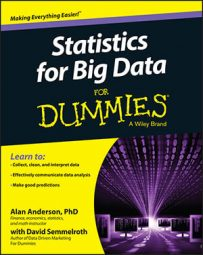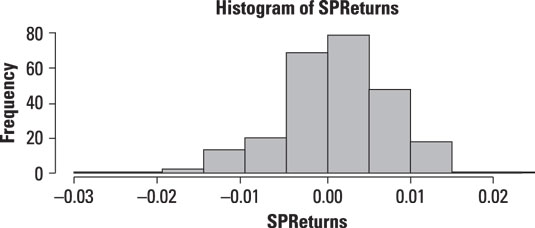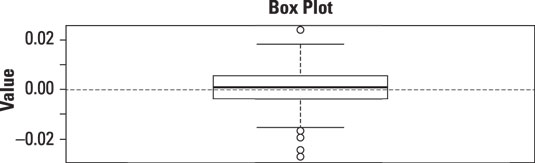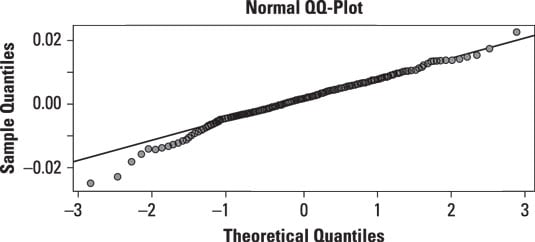##### Statistics for Big Data For DummiesIdentifying data outliers isn't a cut-and-dried matter. There can be disagreement about what does and does not qualify as an outlier. The definition of an outlier depends on the assumed probability distribution of a population. For example, if population really is normally distributed, the graph of a dataset should have the same signature bell shape — if it doesn't, that could be a sign that there are outliers in the data.

You may use three graphical techniques to identify outliers:

• Histograms

• Box plots

• QQ-plots

## Histograms

A histogram is a graph used to visually represent a probability distribution with a series of vertical bars. The horizontal axis shows values or ranges of values for the variable being studied, and the vertical axis shows the corresponding frequencies of these values.

As an example, the Standard and Poor's 500 index (S&P 500) is a stock market index that represents the prices of the 500 largest U.S. stocks, weighted by their market capitalization. A stock's market capitalization equals the price per share times the number of shares outstanding.

The figure shows a histogram of the daily returns for the Standard and Poor's 500 stock market index during the years 2009–2013.A histogram of the daily returns to the S&P 500 from 2009–2013.

According to this histogram, most of the returns were close to zero during this period. Returns above 0.01 (1 percent) or below –0.01 (–1 percent) occurred relatively infrequently. However, for the returns that did occur outside the small range around 0, the occurrence of negative returns outweighed the occurrence of positive returns, as seen by the extreme length of the left tail.

The shape of the histogram shows that the distribution of returns to the Standard and Poor's 500 during this period is unlikely to be normal. One problem is that the normal distribution is symmetrical about its mean, whereas the histogram shows that the distribution of returns is negatively skewed (that is, there's an imbalance between negative and positive returns, with more negative than positive returns).

## Box plots

A box plot shows the distribution of a dataset in a box. The box is based on quartiles, which are like percentiles except that there are only four of them. The box plot is structured as follows:

• The top of the box represents the third quartile (or upper quartile) (Q3) of the data. This is equivalent to the 75th percentile.

• The bottom of the box represents the first quartile (or lower quartile) (Q1) of the data. This is equivalent to the 25th percentile.

• The middle of the box (shown with a line) represents the second quartile (Q2) of the data (also known as the median).

The first quartile of a dataset is a value that is greater than 25 percent of the elements of the dataset and less than the remaining 75 percent. The second quartile (that is, the median) is a value that is greater than 50 percent of the elements and less than the remaining 50 percent. The third quartile is a value that is greater than 75 percent of the elements and less than the remaining 25 percent.

The interquartile range (IQR) is defined as the difference between the third and first quartiles:

IQR = Q3 – Q1

The IQR is used as a measure of dispersion, or how spread out the data is about the center. It can also be used to identify outliers.

For a box plot, there are lines above and below the box. The top line represents the maximum value in a dataset, excluding outliers. The bottom line represents the minimum value in a dataset, again excluding outliers. The individual points shown above and below these lines are the outliers in the dataset.

When you're using a box plot, an outlier is defined as follows:

• If a data point is below Q1 – 1.5(IQR), it is considered to be an outlier.

• If a data point is above Q3 + 1.5(IQR), it is considered to be an outlier.

The following figure shows a box plot of the daily returns to the S&P 500 stock market index during the years 2009–2013.A box plot of the daily returns to the S&P 500 from 2009–2013.

The box plot shows that there is one outlier that is significantly greater than the rest of the returns in the dataset. There are also four outliers that are significantly smaller than the rest of the returns in the dataset. The existence of these outliers shows that the dataset may not be normally distributed.

## QQ-plots

You can plot sample data with a QQ-plot (short for quantile-quantile plot). This plot compares the quantiles of the sample data with the quantiles of a specified probability distribution, such as the normal.

Quantiles are used to divide a dataset into equally sized groups based on the value of a particular numeric variable. There are several types of quantiles, including the following:

• Percentiles divide a dataset into 100 equal groups, each corresponding to a percentage of the total. For example, if a group of 1,000 students takes a standardized exam, and 200 of them receive a score below 300, then 300 would be the 20th percentile of this dataset. This indicates that 20 percent of students scored below 300, whereas the remaining 80 percent scored higher than 300.

• Deciles divide a dataset into ten equal groups, each representing 10 percent of the total. For example, the 4th decile corresponds to the 40th percentile.

• Quartiles divide a dataset into four equal groups, each representing 25 percent of the total. For example, the 3rd quartile corresponds to the 75th percentile.

The following figure shows a QQ-plot of the daily returns to the S&P 500 stock market index during 2009–2013, compared with the normal distribution:A QQ-plot of the daily returns to the S&P 500 from 2009–2013.

The solid line on the graph represents the quantiles of the normal distribution. 0 represents the mean; therefore, half of the values are below 0, and half are above it. About 95 percent of the values are below 2 (2 represents two standard deviations above the mean), whereas 5 percent of the values are below –2 (–2 represents two standard deviations below the mean). If the S&P returns were normally distributed, their quantiles should lie on the line.

The points on the graph are the actual observations in the S&P 500 dataset. For the normal quantiles that are greater than 2 (that is, two standard deviations above the mean), the S&P 500 returns are above the line, which indicates that the right tail is too "fat" to be consistent with the normal distribution. For normal quantiles that are below –1 (that is, one standard deviation below the mean), the S&P 500 returns are below the line, which indicates that the left tail is also too fat to be consistent with the normal distribution.

Overall, the distribution of returns to the S&P 500 appears to be a fat-tailed distribution, meaning that extreme outcomes are much more likely than would be the case with the normal distribution.